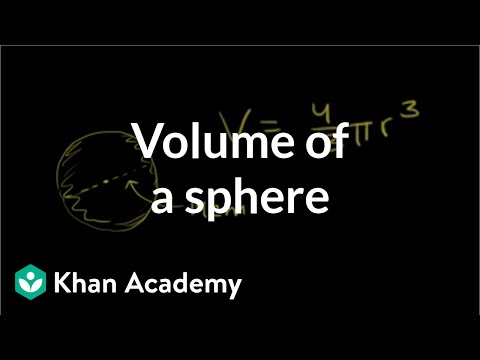Video

# Cylinder volume & surface area (Full video)

Description: Learn how to use the volume and surface area formulas for a cylinder to solve an example problem. Created by Sal Khan. Let's find the volume of a few more solid figures and then if we have time, we might be able to do some surface area problems. And let's say that the height of my cylinder, h, is equal to 8. And then let's say that the radius of one of the top of my cylinder of my soda can, let's say that this radius over here is equal to 4 centimeters.

### Other videos you might be interested in### Volume of a cone (Full video)